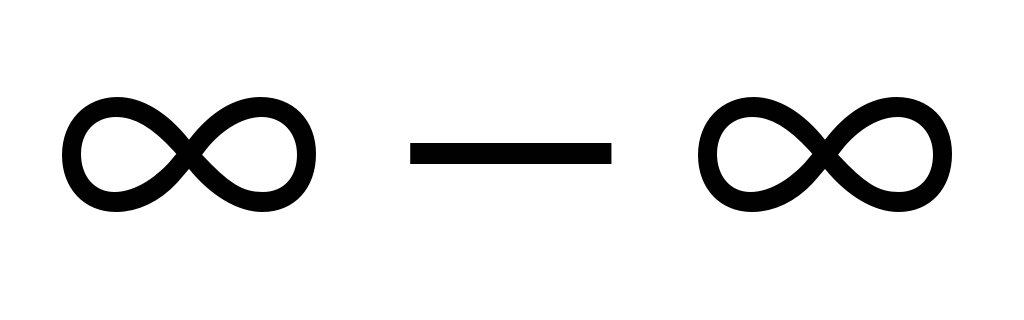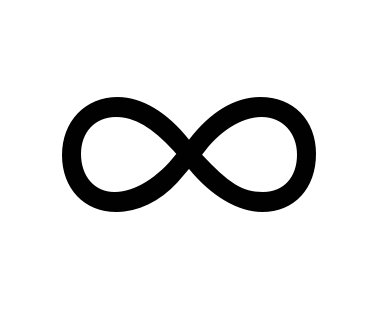# Bachelor of technology (B. Tech) Mechanical/ Electronics/ Computer/ Civil Course Structure First Year

 Page 1/8 Date 05.05.2018 Size 0.95 Mb.
COURSE STRUCTURE & SYLLABUS OF

BACHELOR OF TECHNOLOGY (B.TECH)

Mechanical/ Electrical/ Electronics/ Computer/ Civil
Course Structure First Year

(Common for all streams)
First Semester

 Paper Code Subject BF1 Mathematics – I BF2 Chemistry BF3 English for Communication BF4 Electrical Technology BF5 Mechanics BF6 Introduction to Manufacturing Process

SYLLABUS
BF1 : MATHEMATICS I
1 : LIMITS AND CONTINUITY OF A FUNCTION.
2 : DIFFERENTIATION.

Definition, Derivative by first principle, Differentiation of implicit functions, Differentiation of trigonometric functions, Differentiation of inverse trigonometric functions, Transformation, Differentiation of exponential and Logarithmic Functions, Hyperbolic functions, Derivatives of the inverse hyperbolic functions, Differentiation with respect to a function, Differentiation of Parametric Equations.

3 : SUCCESSIVE DIFFERENTIATION

Calculation of nth derivative, Leibnitz’s theorem.

4 : GENERAL THEOREMS, EXPANSION OF FUNCTIONS.

Rolle’s Theorem, Mean value theorem (Lagrange’s form), Increasing and Decreasing functions, Mean value theorem (Cauchy’s form).

Expansion of functions;

Taylor’s expansion theorem, Maclaurin’s theorem, Taylor’s and Maclaurin’s infinite series.

5 : INDETERMINATE FORM

L’ Hospital’s rule, Evaluation of % form, Evaluation ofform, Evaluation ofform, Evaluation of 00, 1,0 form.